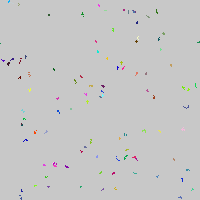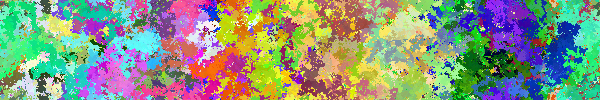# Random Walkers

example processing animation random-walker generative-art random

Note: This example uses parallel arrays. In other words, we’re storing our data across multiple arrays. This is a good way to learn about arrays, but in real life you should use classes instead of parrallel arrays. If you haven’t learned about classes yet, don’t worry about it too much.

This example takes the Random Walker example and uses arrays to have multiple random walkers going at once.

``````int count = 100;

float[] x = new float[count];
float[] y = new float[count];
float[] r = new float[count];
float[] g = new float[count];
float[] b = new float[count];

void setup() {
size(200, 200);

for (int i = 0; i < count; i++) {
x[i] = random(width);
y[i] = random(height);

r[i] = random(256);
g[i] = random(256);
b[i] = random(256);

}

background(200);

noSmooth();
frameRate(1000);
}

void draw(){
for(int i = 0; i < count; i++){
x[i] += random(-1, 1);
y[i] += random(-1, 1);

if(x[i] < 0){
x[i] = width;
}
if(x[i] > width){
x[i] = 0;
}

if(y[i] < 0){
y[i] = height;
}
if(y[i] > height){
y[i] = 0;
}

stroke(r[i], g[i], b[i]);
point(x[i], y[i]);

}
}
``````Code Editor

See the Pen by Happy Coding (@KevinWorkman) on CodePen.

Now that we have this code, we can easily make our program show `1000` random walkers, just by changing the first line:

``````int count = 1000;
``````Or we could modify the walking code so that they move up and down more than left and right:

``````x[i] += random(-.1, .1);
y[i] += random(-1, 1);
``````## Tweak Ideas

• Come up with your own walking logic. Instead of adding `random(-1, 1)`, try adding larger values and then drawing a line from the current position to the next position.
• Base the random movement off of a heading (an angle) that you randomly change. This will result in smoother movement.